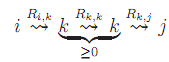## Construct a regular expression, Theory of Computation

Assignment Help:

Given any NFA A, we will construct a regular expression denoting L(A) by means of an expression graph, a generalization of NFA transition graphs in which the edges are labeled with regular expressions rather than just symbols in Σ∪{ε}. We will explain the algorithm using the example of Figure 1.

We begin by adding a new start state s and ?nal state f to the automaton and by extending it to include an edge between every state in Q∪{s} to every state in Q ∪ {f}, including self edges on states in Q. We then consolidate all the edges from a state i to a state j into a single edge, labeled with a regular expression that denotes the set of strings of length 1 or less leading directly from state i to state j in the original automaton. If there was no path directly from i to j in the original automaton the label is ∅. If there were multiple edges (or edges labeled with multiple symbols) the label is the ‘+' of the symbols on those edges (as in the edge from 2 to 1 in the example). There will be an edge from s labeled ε to the original start state and one labeled ∅ to every other state other than f. Similarly, there will be an edge labeled ε from each state in F in the original automaton to state f and one labeled ∅ from those in Q-F to f. The expression graph for the example automaton is given in the right hand side of the ?gure.

The idea, now, is to systematically eliminate the nodes of the transition graph, one at a time, by adding new edges that are equivalent to the paths through that state and then deleting the state and all its incident edges. In general, suppose we are working on eliminating node k. For each pair of states i and j (where i is neither k nor f and j is neither k nor s) there will be a path from i to j through k that looks like:#### Automata and compiler, Automata and Compiler (1) [25 marks] Let N be the...

Automata and Compiler (1) [25 marks] Let N be the last two digits of your student number. Design a finite automaton that accepts the language of strings that end with the last f

#### Production, How useful is production function in production planning?

How useful is production function in production planning?

#### Finite automata, design an automata for strings having exactly four 1''s

design an automata for strings having exactly four 1''s

#### Transition graph for the automaton, Lemma 1 A string w ∈ Σ* is accepted by ...

Lemma 1 A string w ∈ Σ* is accepted by an LTk automaton iff w is the concatenation of the symbols labeling the edges of a path through the LTk transition graph of A from h?, ∅i to

#### Local suffix substitution closure, The k-local Myhill graphs provide an eas...

The k-local Myhill graphs provide an easy means to generalize the suffix substitution closure property for the strictly k-local languages. Lemma (k-Local Suffix Substitution Clo

#### Kleene closure, So we have that every language that can be constructed from...

So we have that every language that can be constructed from SL languages using Boolean operations and concatenation (that is, every language in LTO) is recognizable but there are r

#### Regular expression, what is regular expression?

what is regular expression?

#### Generalization of the interpretation of local automata, The generalization ...

The generalization of the interpretation of strictly local automata as generators is similar, in some respects, to the generalization of Myhill graphs. Again, the set of possible s

#### Assignment, Consider a water bottle vending machine as a finite–state autom...

Consider a water bottle vending machine as a finite–state automaton. This machine is designed to accept coins of Rs. 2 and 5 only. It dispenses a single water bottle as soon as the

#### Numerical integration, what problems are tackled under numerical integratio...

what problems are tackled under numerical integration